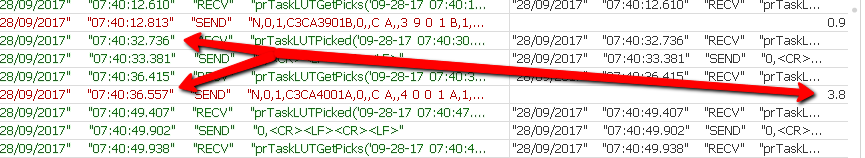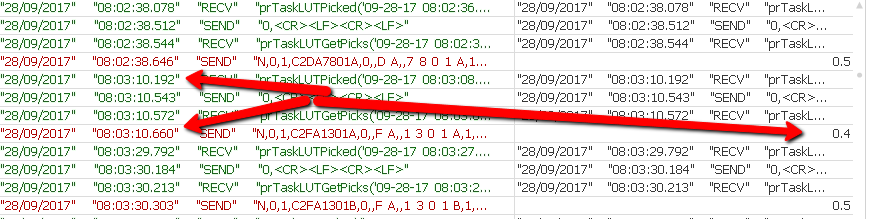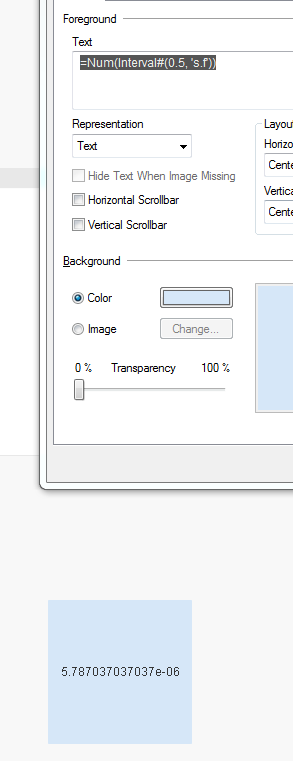Announcements
The way to achieve your own success is the willingness to help somebody else. Go for it!
cancel
Showing results for
Did you mean:Master II

## Expression Issue

Hi All,

I have the following expression.

IF(INTERVAL(\$(vTest1) - above(above(above(\$(vTest1) ))),'s.f') > \$(InitTime), INTERVAL(\$(vTest1) - above(above(above(\$(vTest1) ))),'s.f'), LogFile)

This returns a number for the time difference between transactions, so for one example it returns 3.6 (3.6 Seconds) otherwise it returns the string from the log file - which is working okThe problem I have is that it returns all times, even though my variable \$(InitTime) is set to 0.5, So I should not see 0.4, but I am seeing times less than my Variable any ideas?

1 Solution

Accepted SolutionsMVP

Or this

IF(INTERVAL(\$(vTest1) - above(above(above(\$(vTest1) ))),'s.f') > Interval#(\$(InitTime), 's.f'),

INTERVAL(\$(vTest1) - above(above(above(\$(vTest1) ))),'s.f'), LogFile)

12 RepliesMVP

Where do you seen 0.4 in the image?Master II
Author

It's further down the chart, here is the image scrolled downMaster II
Author

If the time is less than 0.5 then green otherwise red for the line plus the 3 lines aboveMaster II
Author

Hi Sunny, I know what the issue is

if I type the following expression

TIME(0.00000579,'hh:mm:ss.fff') it returns 00:00:00.500.

I am setting my variable to be .5 (500 Milliseconds), so I need to convert 0.5 back to Milliseconds

I will try that now.MVP

Try this

IF(INTERVAL(\$(vTest1) - above(above(above(\$(vTest1) ))),'s.f') > Interval#(\$(InitTime)*2, 's')/2,

INTERVAL(\$(vTest1) - above(above(above(\$(vTest1) ))),'s.f'), LogFile)MVP

Or this

IF(INTERVAL(\$(vTest1) - above(above(above(\$(vTest1) ))),'s.f') > Interval#(\$(InitTime), 's.f'),

INTERVAL(\$(vTest1) - above(above(above(\$(vTest1) ))),'s.f'), LogFile)Master II
Author

No that does not return the correct result.

I need to convert 0.5 to seconds, as Qlikview treats this as 12:00:00.000,

If I set my variable to 0.00000579, it works perfectly, but I want to convert 0.5's as a numberMVP

That is what it should be doing... this give me

=Num(Interval#(0.5, 's.f'))

thisReplace 0.5 with your variable and it should work (I think)Master II
Author

Sorry it's working thanksCommunity Browser